Ex 4.4

Chapter 4 Class 12 Determinants
Serial order wise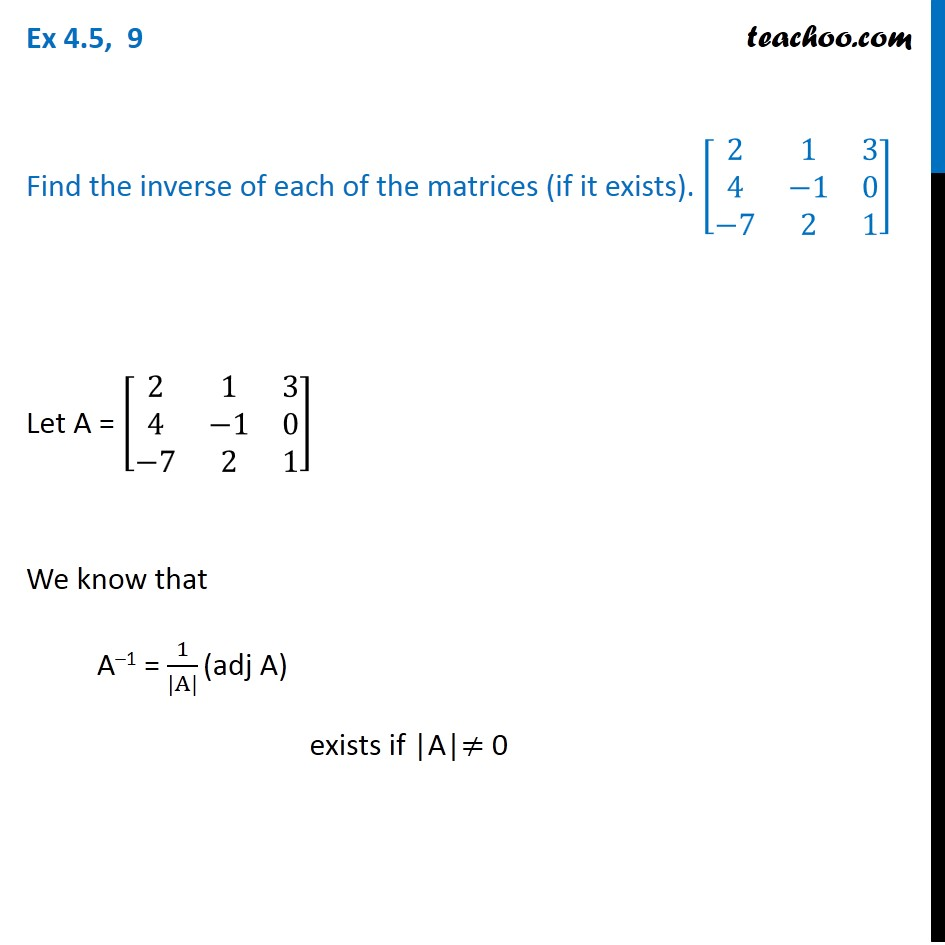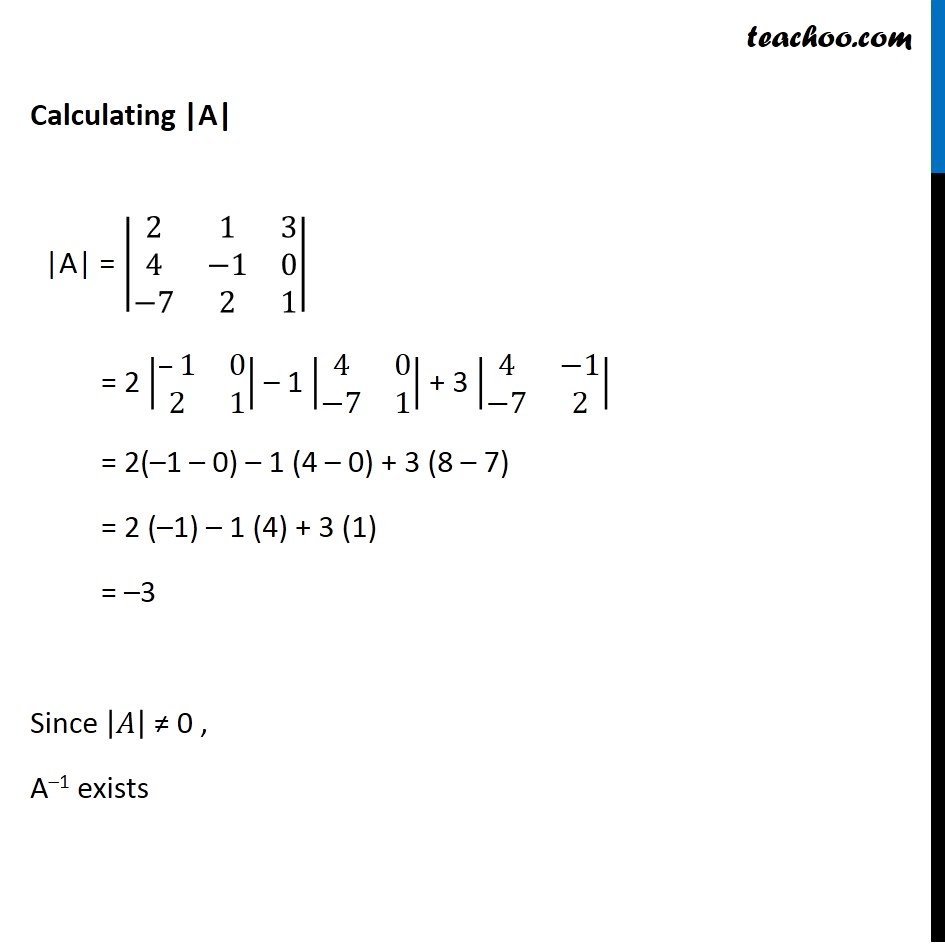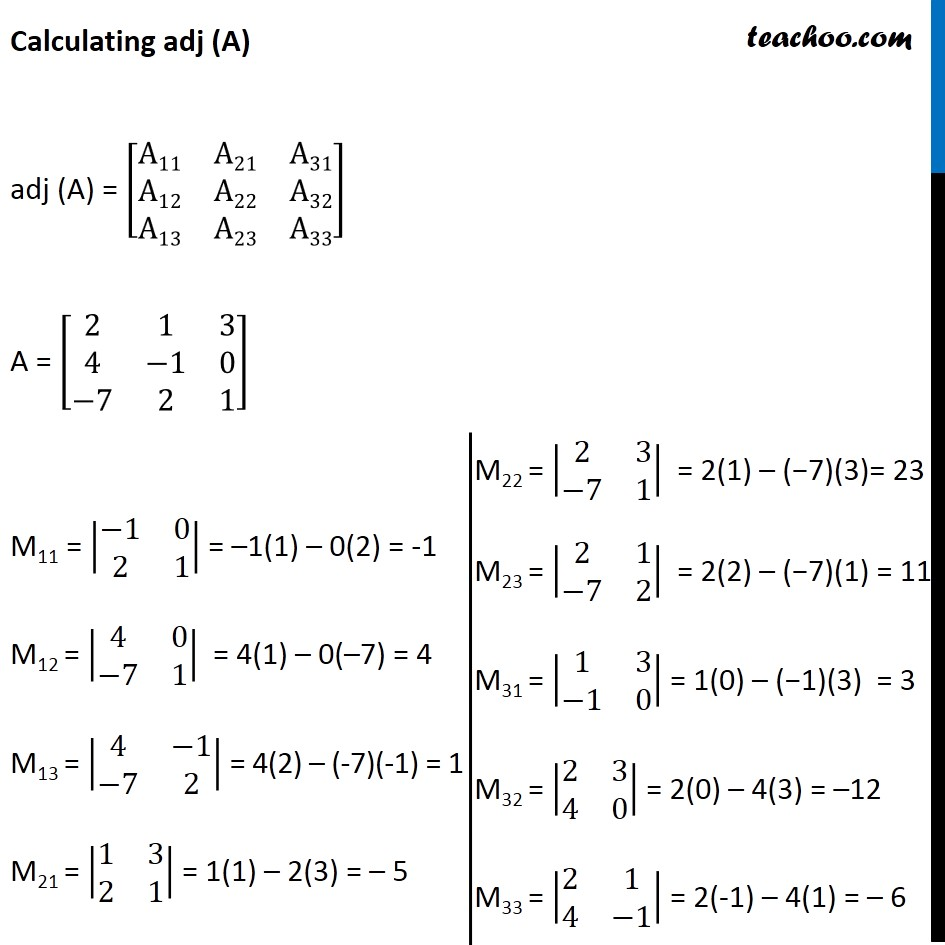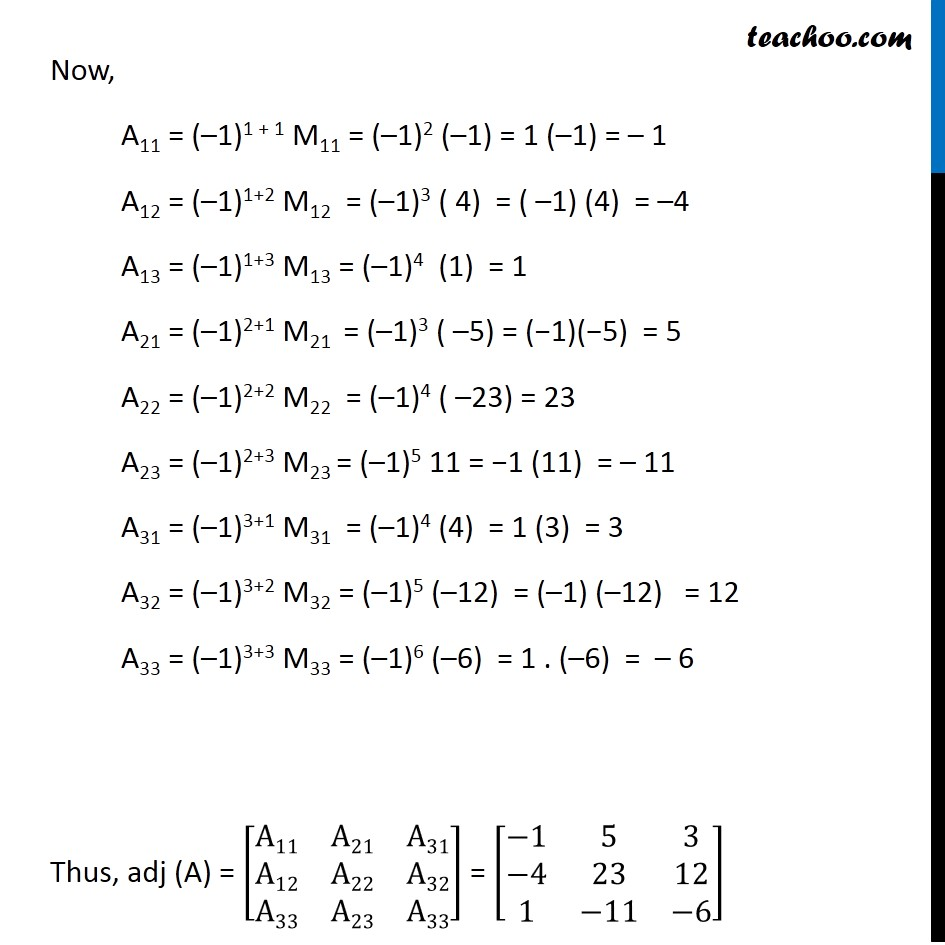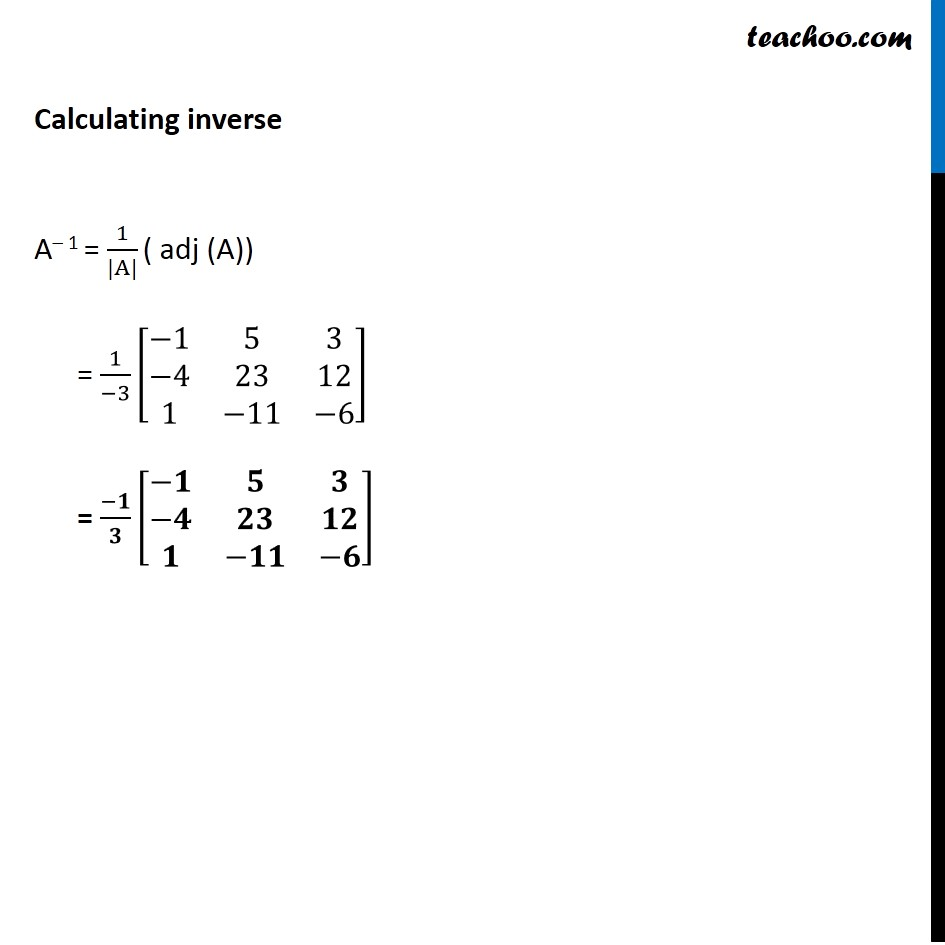Learn in your speed, with individual attention - Teachoo Maths 1-on-1 Class

### Transcript

Ex 4.4, 9 Find the inverse of each of the matrices (if it exists). [■8(2&1&[email protected]&−1&0@−7&2&1)] Let A = [■8(2&1&[email protected]&−1&0@−7&2&1)] We know that A–1 = 1/(|A|) (adj A) exists if |A|≠ 0 Calculating |A| |A| = |■8(2&1&[email protected]&−1&0@−7&2&1)| = 2 |■8(–1&[email protected]&1)| – 1 |■8(4&0@−7&1)| + 3 |■8(4&−1@−7&2)| = 2(–1 – 0) – 1 (4 – 0) + 3 (8 – 7) = 2 (–1) – 1 (4) + 3 (1) = –3 Since |𝐴| ≠ 0 , A–1 exists Calculating adj (A) adj (A) = [■8(A11&A21&[email protected]&A22&[email protected]&A23&A33)] A = [■8(2&1&[email protected]&−1&0@−7&2&1)] M11 = |■8(−1&[email protected]&1)| = –1(1) – 0(2) = -1 M12 = |■8(4&0@−7&1)| = 4(1) – 0(–7) = 4 M13 = |■8(4&−1@−7&2)| = 4(2) – (-7)(-1) = 1 M21 = |■8(1&[email protected]&1)| = 1(1) – 2(3) = – 5 M22 = |■8(2&3@−7&1)| = 2(1) – (−7)(3)= 23 M23 = |■8(2&1@−7&2)| = 2(2) – (−7)(1) = 11 M31 = |■8(1&3@−1&0)| = 1(0) – (−1)(3) = 3 M32 = |■8(2&[email protected]&0)| = 2(0) – 4(3) = –12 M33 = |■8(2&[email protected]&−1)| = 2(-1) – 4(1) = – 6 Now, A11 = (–1)1 + 1 M11 = (–1)2 (–1) = 1 (–1) = – 1 A12 = (–1)1+2 M12 = (–1)3 ( 4) = ( –1) (4) = –4 A13 = (–1)1+3 M13 = (–1)4 (1) = 1 A21 = (–1)2+1 M21 = (–1)3 ( –5) = (−1)(−5) = 5 A22 = (–1)2+2 M22 = (–1)4 ( –23) = 23 A23 = (–1)2+3 M23 = (–1)5 11 = −1 (11) = – 11 A31 = (–1)3+1 M31 = (–1)4 (4) = 1 (3) = 3 A32 = (–1)3+2 M32 = (–1)5 (–12) = (–1) (–12) = 12 A33 = (–1)3+3 M33 = (–1)6 (–6) = 1 . (–6) = – 6 Thus, adj (A) = [■8(A11&A21&[email protected]&A22&[email protected]&A23&A33)] = [■8(−1&5&3@−4&23&[email protected]&−11&−6)] Calculating inverse A– 1 = 1/(|A|) ( adj (A)) = 1/(−3) [■8(−1&5&3@−4&23&[email protected]&−11&−6)] = (−𝟏)/𝟑 [■8(−𝟏&𝟓&𝟑@−𝟒&𝟐𝟑&𝟏𝟐@𝟏&−𝟏𝟏&−𝟔)]